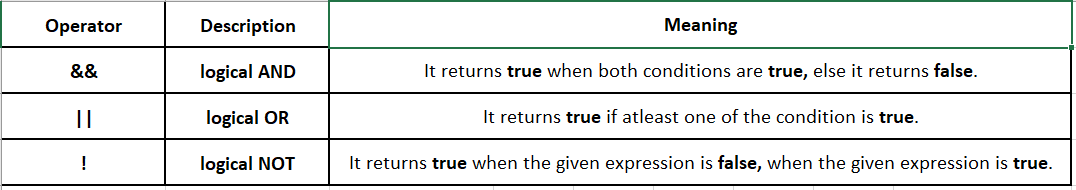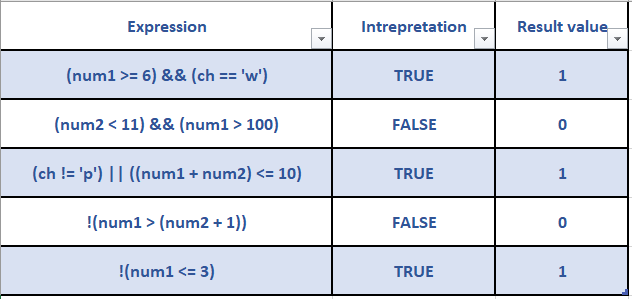In this tutorial, we are going to see Logical Operators in C Language.

## Logical Operators:

Logical operators are used to performing logical operations on the given expressions.

An expression containing a logical operator returns either 0 or 1 depending on the evaluation of the expression to either false or true respectively.

In Cfalse is represented as 0 (zero) and all non-zero values can be treated as true.

Given below are three logical operators in C.According to the coding conventions in C, a single space should be provided to the left and to the right of the operator.

The below table demonstrates the use of various relational and equality operators using variables

``````int num1 = 7;
float num2 = 5.5;
char ch = 'w';``````